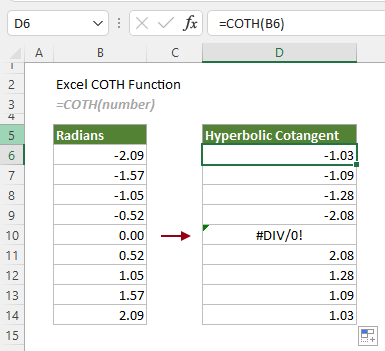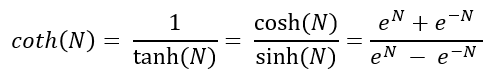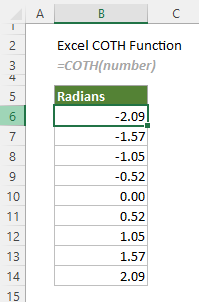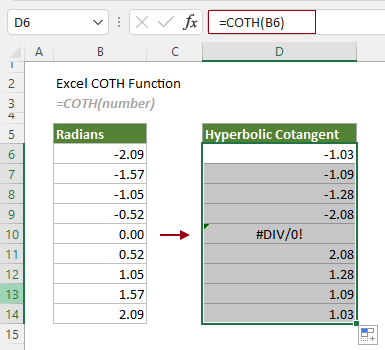Note: The other languages of the website are Google-translated. Back to English

## Excel COTH function

The COTH function returns the hyperbolic cotangent of a hyperbolic angle in radians.

Note: This function is available in Excel for Microsoft 365, Excel 2013 and later versions.=COTH(number)

#### Arguments

Number (required): The angle in radians for which you want to calculate the hyperbolic cotangent.

#### Remarks

1) The #VALUE! error occurs if “number” is non-numeric.
2) The #DIV/0! error occurs if “number” is equal to zero.
3) The #NUM! error occurs if one of the following conditions is met:
-- “number” is less than -2^27
Or
-- “number” is greater than 2^27
4) If your angles are in degrees, you need to convert them to radians by using the RADIANS function:
5) The equation for the COTH function is as follows:#### Return value

It returns a hyperbolic cotangent value.

#### Example

To calculate the hyperbolic cotangent of the angles in radians listed in the table below, the COTH function can do you a favor.Select a blank cell, such as D6 in this case, enter the following formula and press the Enter key to get the first result. Select the first result cell and drag its AutoFill Handle down to get the rest of the results.

=COTH(B6)Notes:

1) The formula above uses a cell reference as the argument, you can type the radians in the formula instead. The formula in the cell D6 can be changed to:
=COTH(-2.09)
2) If the supplied angles are in degrees, please convert them to radians before supplying to the COTH function.
You can apply the RADIANS function to convert a degree number to radians:
Or embed the RADIANS function directly into the COTH function, such as:

#### Related Functions

Excel COSH Function
The COSH function returns the hyperbolic cosine of a given number.

Excel ACOSH Function
The ACOSH function returns the inverse hyperbolic cosine of a number.

Excel COT Function
The COT function is used to calculate the cotangent of an angle in radians.

Excel ACOT Function
The ACOT function calculates the arccotangent (inverse cotangent) of a number, and returns an angle, in radians, in the range from 0 (zero) to π (pi).

Excel ACOTH Function
The ACOTH function returns the inverse hyperbolic cotangent of a number.

### The Best Office Productivity Tools

#### Kutools for Excel - Helps You To Stand Out From Crowd

Would you like to complete your daily work quickly and perfectly? Kutools for Excel brings 300 powerful advanced features (Combine workbooks, sum by color, split cell contents, convert date, and so on...) and save 80% time for you.

• Designed for 1500 work scenarios, helps you solve 80% Excel problems.
• Reduce thousands of keyboard and mouse clicks every day, relieve your tired eyes and hands.
• Become an Excel expert in 3 minutes. No longer need to remember any painful formulas and VBA codes.
• 30-day unlimited free trial. 60-day money back guarantee. Free upgrade and support for 2 years.#### Office Tab - Enable Tabbed Reading and Editing in Microsoft Office (include Excel)

• One second to switch between dozens of open documents!
• Reduce hundreds of mouse clicks for you every day, say goodbye to mouse hand.
• Increases your productivity by 50% when viewing and editing multiple documents.
• Brings Efficient Tabs to Office (include Excel), Just Like Chrome, Firefox, And New Internet Explorer.